# Class 12 Chemistry Important Questions – Term 1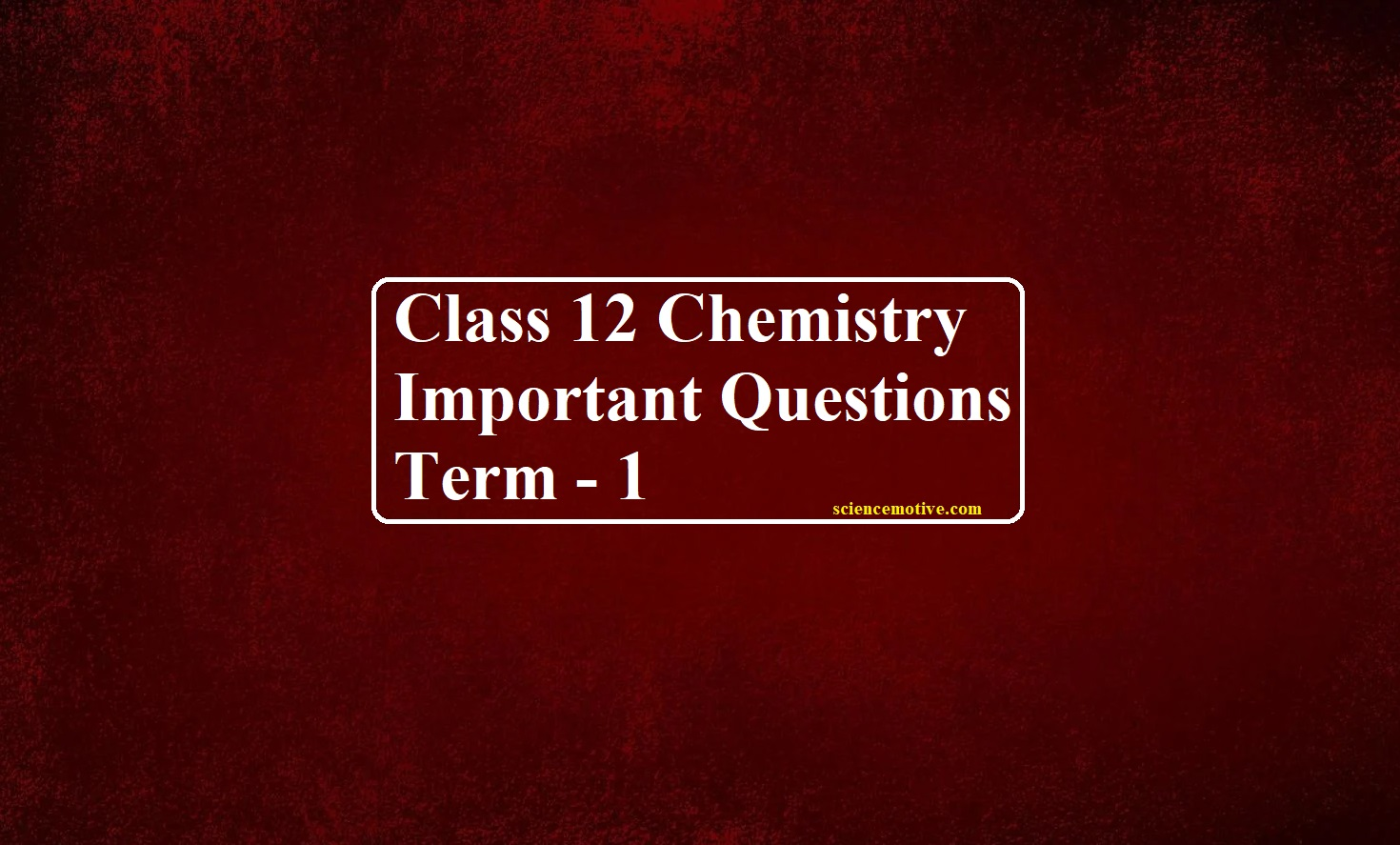# Class 12 Chemistry Important Questions

Class 12 Chemistry Important Questions

Que 1. Analysis shows that nickel oxide has the formula 0.98 NiO. What fraction of the Nickel exists as Ni2+ and Ni3+ ions respectively?
(a) 90%, 10%              (b) 85%, 15%              (c) 96%, 4%                (d) 76%, 24%
Ans 1. (c)
According to the statements
Formula is Ni0.98O1.00
So, the ratio of Ni:O = 98:100
So, if there are 100 atoms of oxygen then 98 atoms of Ni
Let number of atoms of Ni+2 = x
Then number of atoms of Ni+3 = 98–x
Charge on Ni = charge on O
So that oxygen has charge –2
3(98–x) + 2x = 2 (100)
294 – 3x + 2x = 200
–x = – 94
⸫ x = 94
Percentage of Ni+2 = (atom of Ni+2 / total number of atoms of Ni)100
=100 (94/98)
= 96%

Que 2. Gold (Au) crystallizes in cubic close-packed (FCC). The atomic radius of gold is 144 pm and the atomic mass of Au = 197.0 amu. The density of Au is:
(a) 19.4 g cm–3            (b) 194 g cm–3             (c) 39.4 g cm–3                        (d) 0.194 g cm–3
Ans 2. (a)
We know for FCC unit cell r = a/2√2
a = 2 √2r = 2×1.414×0.144nm = 0.407 nm = 0.407×10-7 cm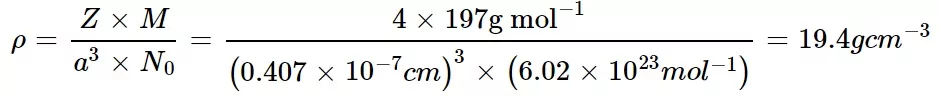Que 3. An alloy of copper, silver, and gold is found to have copper constituting the ccp lattice. If silver atoms occupy the edge centres and gold is present at the body centre, the alloy will have the formula:
(a) Cu4 Ag4 Au           (b) Cu Ag Au              (c) Cu4 Ag2 Au           (d) Cu4 Ag3 Au
Ans 3. (d)
Number of Cu atoms at corners = 8 × 1/8
Number of Ag atoms at edge centres = 12 × ¼
Number of Au atoms at body centre =1×1=1
∴ The formula is Cu4Ag3Au.

Que 4. Pick out the incorrect statement:
(a) NaCl has 8:8 coordination, while CsCl is with 6:6 coordination
(b) In Na2O each oxide ion is coordinated by 8 Na+ ions and each Na+ ion by 4 oxide ions
(c) NaCl structure transforms to CsCl structure on heating
(d) In the CaF2 structure, each F ion is coordinated by 4 Ca2+ ions and each Ca2+ ion is coordinated by 8 F ions
Ans 4. (a) Na+ = 6, Cl = 6,

Que 5. A compound has a hexagonal closed packed structure what is the total number of voids in 0.5 mol of it?
(a) 6.02 × 1023             (b) 9.33 × 1023            (c) 12.04 × 1023                       (d) 3.01 × 1023
Ans 5. (b) 9.33 × 1023
No of atoms present in 0.5 moles of a compound are
No of atoms = 0.5 × Avogadro’s number
No of atoms = 0.5 × 6.022 × 1023 atoms
We know for n atoms there are n Octahedral voids
⸫ Octahedral voids = 3.011 × 1023 voids
We know for n atoms there are 2n Tetrahedral voids.
Tetrahedral voids = 2 × no of atoms
Tetrahedral voids = 2 × 3.011 × 1023
Tetrahedral Voids = 6.022 × 1023 voids
⸫ Total no of Voids = Tetrahedral Voids + Octahedral Voids
Total voids = 6.022 × 1023 + 3.011 × 1023
Total voids = 9.033 × 1023 voids

Class 12 Chemistry Important Questions

Que 6. A colligative property is not represented by:
(a) Elevation in boiling point                                     (b) Osmotic pressure
(c) Optical activity                                                      (d) Relative lowering of vapour pressure
Ans 6. (c) Optical activity

Que 7. Which of the following 0.1 M aqueous solutions will have the lowest freezing point?
(a) K2SO4                    (b) NaCl                      (c) Urea                       (d) Glucose
Ans 7. (a) K2SO4
Urea and glucose do not dissociate in solution. Sodium chloride gives two ions and potassium sulphate gives three ions per formula unit.
Therefore, the effective number of particles is maximum in potassium sulphate, and it shows the maximum depression in freezing point.

Que 8. The molality of 1 litre solution of 93% H2SO4 (w/v) having density 1.84 g/mL is:
(a) 10.43                      (b) 1.043                     (c) 0.1043                    (d) 100.43
Ans 8. (a) 10.43 m

Que 9. To 500 cm3 of water, 3.0 × 10–3 kg of acetic acid is added. If 23% of acetic acid is dissociated, what will be the depression in freezing point? Kf and density of water at 1.86 K kg–1 and 0.0997 g cm–3 respectively.
(a) 0.186 K                  (b) 0.228 K                 (c) 0.371 K                  (d) 0.555 K
Ans 9. (b) 0.228 K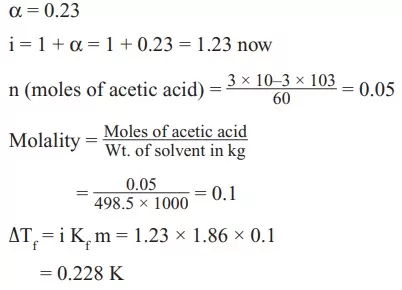Que 10. Which of the following statement is true about ideal solutions?
(a) The volume of mixing is zero                               (b) The enthalpy of mixing is zero
(c) Both A and B                                                        (d) None of these
Ans 10. (c) Both A and B

Class 12 Chemistry Important Questions

Que 11. Which one of the statements given below-concerning the properties of solutions, describe a colligative effect?
(a) Boiling point of pure water decreases by the addition of ethanol
(b) Vapour pressure of pure water decreases by the addition of nitric acid
(c) Vapour pressure of pure benzene decreases by the addition of naphthalene
(d) Boiling point of pure benzene increases by the addition of toluene
Ans 11. (c) Vapour pressure of pure benzene decreases by the addition of naphthalene
Vapour pressure of pure benzene decreases by the addition of naphthalene. Naphthalene is non -volatile in nature so it decreases the vapour pressure of benzene. The addition of a non-volatile solute always lowers the vapour pressure.

Que 12. The bonds present in N2O5 are:
(a) Only ionic                                                              (b) Covalent and coordinate
(c) Only covalent                                                        (d) Covalent and ionic
Ans 12. (b) Covalent and coordinate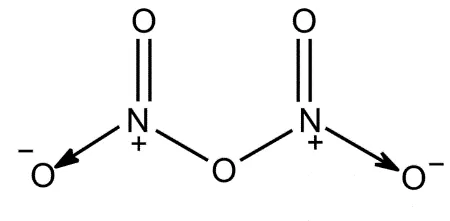Que 13. What is formed when 3 parts of HCl is mixed with one part of HNO3?
(a) NOCl                     (b) NH4Cl                   (c) NCl3                       (d) NO
Ans 13. (a) NOCl
3 HCl(aq) + HNO3(aq) → NOCl(g) + Cl2(g) + 2 H2O

Que 14. Which of the following is used in smoke screens?
(a) HCl                        (b) PH3                        (c) NH3 + HCl             (d) NH3
Ans 14. (b) PH3

Que 15. Sugarcane on reaction with nitric acid gives
(a) 2HCOOH (two moles)                                          (b) CO2 and SO2
(c) no reaction                                                             (d) (COOH)2
Ans 15. (d) (COOH)2
[2 HNO3 → H2O + 2 NO2 + O] × 18
C12H22O11 + 18 O → 6 (COOH)2 + 5 H2O
Final Equation:         C12H22O11 + 36 HNO3 → 6 (COOH)2 + 36 NO2 + 23 H2O

Class 12 Chemistry Important Questions

Que 16. What are the products obtained when ammonia is reacted with excess chlorine?
(a) N2 and NH4Cl        (b) N2 and NCl3          (c) NCl3 and HCl                    (d) N2 and HCl
Ans 16. (c) NCl3 and HCl
NH3 + 3Cl2 → NCl3 + 3HCl
NCl3 is reactive and act as an explosive compound.

Que 17. The gas O3 (ozone) cannot oxidise
(a) KI                          (b) FeSO4                    (c) KMnO4                              (d) K2MnO4
Ans 17. (c) KMnO4
Because Mn is already at its highest oxidation state i.e. + 7.

Que 18. When SO2 is passed through acidified K2Cr2O7 solution
(a) The solution is decolourised                                   (b) The solution is turned blue
(c) Green Cr2(SO4)3 is formed                                    (d) SO2 is reduced
Ans 18. (c) Green Cr2(SO4)3 is formed. During the reaction orange colour of K2Cr2O7 changes to green due to the formation of Cr2(SO4)3 when SO2 passed.
K2​Cr2​O7​ + 3 SO2 ​+ H2​SO4 ​→ K2​SO4 ​+ Cr2​(SO4​)3 ​+ H2​O

Que 19. The incorrect order of bond angles (smallest first) in H2S, NH3, BF3, and SiH4 is
(1) H2S < NH3 < BF3 < SiH4
(2) H2S < NH3 < SiH4 < BF3
(3) NH3 < H2S < SiH4 < BF3
(4) H2S < SiH4 < NH3 < BF3
(a) 1, 2, 3                     (b) 2, 3, 4                    (c) 1, 3, 4                     (d) 1, 2, 4
Ans 19. (c) 1, 3, 4
Species                       lp                    bp                               VSEPR              bond angle
H2S                              2                      2                                  lp-lp                900
NH3                             1                      3                                  bp-bp               1070
BF3                              0                      3                                  bp-bp               1200
SiH4                            0                      4                                  bp-bp               109.280

Que 20. SO2 acts as a temporary bleaching agent but Cl2 acts as a permanent bleaching agent. Why?
(a) Cl2 bleaches due to oxidation but SO2 due to reduction
(b) Cl2 bleaches due to reduction but SO2 due to oxidation
(c) Both (a) and (b)
(d) None of these
Ans 20. (a) Cl2 bleaches due to oxidation but SO2 is due to the reduction

Class 12 Chemistry Important Questions

Que 21. Total number of lone pair of electrons in XeOF4 is:
(a) 0                             (b) 1                            (c) 2                             (d) 3
Ans 21. (b) 1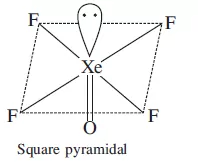Que 22. Chlorobenzene on heating with NH3 under pressure in the presence of cuprous chloride gives
(a) Nitrobenzen           (b) Aniline      (c) Benzamide             (d) o-and p-chloroaminobenzene
Ans 22. (b) Aniline

Que 23. The major product in the reaction CH3 – CH2 –Br with AgCN is
(a) CH3 – CH2 – CN               (b) CH3 – CH2 Ag       (c) CH3 – CH2 NC      (d) None of these
Ans 23. (c) CH3 – CH2 NC

Que 24. Identify the final product (C) in the following sequence of reactions.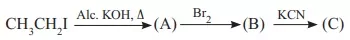(a) NCCH2 CH2 CN                (b) CH3 CH2 Br           (c) CH3 CH2 CN         (d) BrCH2 CH2 CN
Ans 24. (c) CH3 CH2 CN

Que 25. Arrange the following halides in the decreasing order of SN1 reactivity.
1. CH3 CH2 CH2 Cl

2. CH2 = CHCH(Cl)CH3

3. CH3 CH2 CH(Cl)CH3
(a) 1 > 2 > 3                            (b) 2 > 3 > 1                       (c) 2 > 1 > 3                (d) 3 > 2 > 1
Ans 25. (b) 2 > 3 > 1

Que 26. The compound C in the following series of reactions is

Class 12 Chemistry Important Questions

Que 27. Wurtz reaction involves the reduction of an alkyl halide with
(a) HI                          (b) Zn/HCl                  (c) Zn in an inert solvent                    (d) Na in ether
Ans 27. (d) Na in ether

Que 28. Isopropyl chloride undergoes hydrolysis by
(a) SN1 and SN2 mechanisms                          (b) Neither SN1 nor SN2 mechanisms
(c) SN1 mechanism only                                 (d) SN2 mechanism only
Ans 28. (a) SN1 and SN2 mechanisms

Que 29. The molecule which the highest boiling point is:
(a) CH3–CHCl–CH3                                       (b) CH3–CHOH–CH2CH2OH
(c) CH3CH2CH2CH2Cl                                   (d) CH3–CHOH–CH3
Ans 29. (b) CH3–CHOH–CH2CH2OH

Que 30. The correct order of boiling points for primary (1°), secondary (2°) and tertiary (3°) alcohols is:
(a) 1° > 2° > 3°           (b) 3° > 2° > 1°           (c) 2° > 1° > 3°                       (d) 2° > 3° > 1°
Ans 30. (a) 1° > 2° > 3°

(a) Assertion and reason both are correct statements but reason is correct explanation for assertion.
(b) Assertion and reason both are correct statements but reason is not correct explanation for
assertion.
(c) Assertion is incorrect statement but reason is correct.
(d) Assertion is correct statements but reason is incorrect statement.

Que 31. Assertion: Xenon forms fluorides.
Reason: Because 5d orbitals are available for valence shell expansion.
Ans 31. (a)

Que 32. Assertion: When iodine is heated under atmospheric pressure conditions, it transforms to vapour without passing through the liquid state.
Reason: If the triple point pressure of a system is high and unless external pressure is applied to exceed the triple point pressure, sublimation will take place.
Ans 32. (a)

Que 33. Assertion: All solutes become more soluble in water at higher temperatures.
Reason: The amount of solute that dissolves depends upon the mature temperature and pressure (gases) of the substance.
Ans 33. (c)

Que 34. Assertion: Phenol reacts with acyl halides in presence of pyridine to form phenylacetate.
Reason: Benzylation of phenol is carried out in the presence of NH4OH
Ans 34. (d)

Que 35. Assertion: 4-nitrophenol is more acidic than 2,4,6-trinitrophenol.
Reason: Phenol is a weaker acid than carbonic acid.
Ans 35. (c) (A) is incorrect but (R) is correct

Class 12 Chemistry Important Questions

Que 36. Calculate the osmotic pressure of a solution containing 6.00 g urea and 9.00 g glucose in one litre of solution at 27°C.
Ans 36. πV = (n1​ + n2​) RT
n1 = w1/M1 = 9/180 = 1/20 = 0.05
n2 = w2/M2 = 6/60 = 0.5
π × 1 lt = (0.05 + 0.5) × 0.082 × 300
π = 4.92 atm

Que 37. (a) What do you understand by the term that Kf for water is 1.86 Kkg/mol?
(b) State Raoult’s Law for a solution containing volatile components. How does Raoult’s law become a special case of Henry’s Law?
Ans 37. (a) It means that the freezing point of water is lowered by 1.86K when 1mol of a non-volatile solute is dissolved in 1000 g of water.
(b) According to Raoult’s law, the vapour pressure of a component is directly proportional to its mole fraction at a particular temperature.
Pa = Pa0​ Xa
Pb​ = Pb0​ Xb
Ptotal ​ = Pa0​ Xa​ + Pb0​ Xb
In Henry’s law, the mole fraction of a gas at a particular temperature is proportional to the pressure exerted over the gas.
Pgas​ = k Xgas
So, we can say that for the volatile substance it is a special case of Henry’s law.

Que 38. (a) Give the significance of a ‘lattice point’.
(b) Name the parameters that characterize a unit cell.
Ans 38. (a) The significance of a lattice point is that each lattice point represents one constituent particle of a solid which may be an atom, a molecule (group of atoms), or an ion.
(b) The six parameters that characterize a unit cell are as follows.
(i) Its dimensions along the three edges, a, b, and c  These edges may or may not be equal.
(ii) Angles between the edges  These are the angles (between edges b and c), (between edges a and c), and (between edges a and b).
Que 39. (a) 6XeF4 + 12 H2O →
(b) XeF6 + 3 H2O →
(c) XeF6 + 2 H2O → (partial hydrolysis)
Ans 39. (a) 6 XeF4 + 12 H2O → 2 XeO3 + 4 Xe + 3 O2 + 24 HF
(b) XeF6 + 3 H2O → XeO3​ + 6 HF
(c) XeF6 + 2 H2O → (partial hydrolysis) XeF6 ​+ H2​O → XeO2F2​ + 2 HF

Que 40. (a) What are ambident nucleophiles?
(b) Which is a better nucleophile, a bromide ion or iodide ion?
Ans 40. (a) The nucleophiles that can attack through two different sites are known as ambident nucleophiles. For example, cyanide ion is an ambident nucleophile. It can attack through either the C atom or N atom to form alkyl cyanide or alkyl isocyanide.
(b) Nucleophilicity increases as we go down the periodic table. So due to the larger size of iodide ion it has higher polarizability thus, it is a better nucleophile.

Que 41. Explain why?
(a) Alkylhalides, though polar, are immiscible in water.
(b) Grignard reagents should be prepared under anhydrous conditions?
Ans 41. (a) A strong hydrogen bonding exists between the molecules of water. But the new force of attraction between the alkyl halides and water molecules is weaker than the alkyl halide-alkyl halide and water-water forces of attraction. Hence, alkyl halides (though polar) are immiscible with water.
(b) Grignard reagents are very reactive. In the presence of moisture, they react to give alkanes. Therefore, Grignard reagents should be prepared under anhydrous conditions.
RMgX + H2O → R-H + Mg(OH)X

OR

Que 41. (a) Explain why propanol has a higher boiling point than that hydrocarbon, butane?
(b) While separating a mixture of ortho and para nitrophenols by steam distillation, name the isomer which will be steam volatile. Give reason.
Ans 41. (a) Propanol undergoes intermolecular H-bonding because of the presence of the -OH group. On the other hand, butane does not. Thus, extra energy is required to break hydrogen bonds.
(b) Intramolecular H-bonding is present in o-nitrophenol. In p-nitrophenol, the molecules are strongly associated due to the presence of intermolecular bonding. Hence, o-nitrophenol is steam volatile.

Que 42. Give two reactions that show the acidic nature of phenol. Compare the acidity of phenol with that of ethanol.
Ans 42. Reaction (i) Phenol reacts with sodium to give sodium phenoxide, liberating H2.
Reaction (ii) Phenol reacts with sodium hydroxide to give sodium phenoxide and water as by-products. The acidity of phenol is more than that of ethanol.

Que 43. Niobium crystallizes in a body-centred cubic structure. If density. If the density is 8.55 g/cm, calculate the atomic radius of niobium using its atomic mass of 93 u.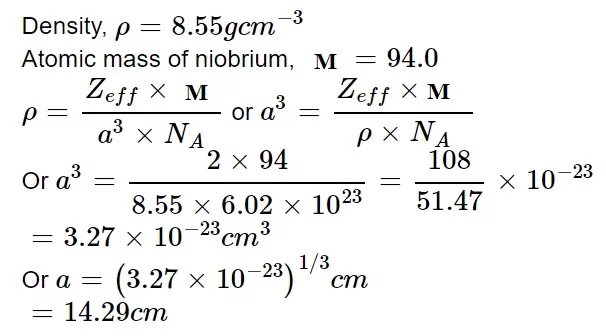Que 44. (a) Out of 1 molal and 1 molar aqueous solution which is more concentrated? Justify.
(b) Why is freezing point depression of 0.1 M sodium chloride solution nearly twice that of 0.1 M glucose solution?
Ans 44. (a) 1 molar aqueous solution is more concentrated than 1 molal aqueous solution because 1 molar solution contains 1 mole of solute in 1 litre of the solution which includes both solute and solvent. So, the mass of solvent (i.e. water) is less than 1000 grams.

(b) NaCl is an electrolyte and it dissociates completely whereas glucose being a non-electrolyte does not dissociate. Hence, the number of particles in 0.1 M NaCl solution is nearly double for NaCI solution than that for glucose solution of the same molarity.

Que 45. (a) With what neutral molecules is ClO is isoelectronic? Is that molecule a Lewis base?
(b) Why are halogens coloured?

Ans 45. (a)  ClO is isoelectronic to ClF as both the compounds contain 26 electrons in all. Yes, ClF Molecule is a Lewis base as it accepts electrons from F to form ClF3.
(b) The colour of halogens is due to the absorption of different quanta of radiations in the visible region which results in excitation of outer electrons to higher energy levels, thus different colours are observed.

Que 46. (a) Why is sulphuric acid not used during the reaction of alcohols with KI?
(b) Do the following conservation
(i) Methyl bromide to acetone
(ii) Benzyl-chloride to 2- phenylacetic acid
(c) Which compound in each of the following pairs will react faster in SN2 reaction with OH and why?
(i) CH3Br or CH3I                                           (ii) (CH3)3 CCl or CH3Cl
Ans 46. (a) During the reaction of alcohol with KI In the presence of sulphuric acid (H2SO4), KI produces HI
2KI + H2SO4     →    2KHSO4 + 2HI
Since H2SO4 is an oxidizing agent, it oxidizes HI (produced in the reaction to I2).
2HI + H2SO4    → I2 + SO2 + H2O
As a result, the reaction between alcohol and HI to produce alkyl iodide cannot occur. Therefore, sulphuric acid is not used during the reaction of alcohols with KI.
⸫ In place of H2SO4 non-oxidising acid such as H3PO4.
(b) Conversions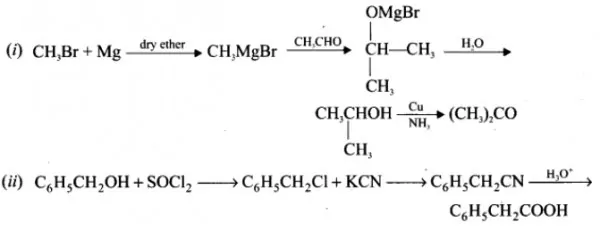(c) As CH3I provides less hindrance, it will react faster by SN2 reaction than CH3Br. CH3Cl being the primary halide provides less hindrance to the action of OH-, thus it will react by SN2 more easily than (CH3)3CCl.

Class 12 Chemistry Important Questions